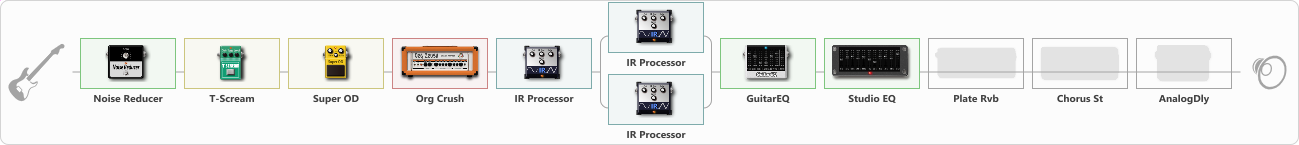# Metallica (Black Album)

Discussion in 'ToneLib-GFX presets' started by Tony the Metalhead, Dec 6, 2021.

1. ### Tony the MetalheadActive Member

Metallica (Black Album)

Preset name: Metallica (Black Album)

Updated

Effects chain:Effect: "Noise Reducer" (Dynamics / Filter), active - "yes"
{
"Sens" = 65
"Mode" = Hard
}

Effect: "T-Scream" (Overdrive / Distortion), active - "yes"
{
"Drive" = 5
"Tone" = 40
"Level" = 10
}

Effect: "Super OD" (Overdrive / Distortion), active - "yes"
{
"Drive" = 60
"Tone" = 60
"Level" = 40
}

Effect: "Org Crush" (Amp simulators), active - "yes"
{
"Gain" = 80
"Bass" = 15
"Middle" = 5
"Treble" = 66
"Presence" = 65
"Master" = 30
"Level (dB)" = 9
}

Effect: "IR Processor" (Cabinets), active - "yes"
{
"IR" = Black_LR_Stereo
"Low Cut (Hz)" = 175
"Hi Cut (kHz)" = 20.0
"Mix" = 100
"Level (dB)" = 0
}

Effect: "Splitter" (Dynamics / Filter)
{
"A-Bypass" = Off
"A-Pan" = 0
"A-Level" = 55
"B-Bypass" = Off
"B-Pan" = 0
"B-Level" = 55

'A' branch:
{

Effect: "IR Processor" (Cabinets), active - "yes"
{
"IR" = OD-R112-V30-SM58-P05-20
"Low Cut (Hz)" = 80
"Hi Cut (kHz)" = 20.0
"Mix" = 100
"Level (dB)" = 0
}
}
'B' branch:
{

Effect: "IR Processor" (Cabinets), active - "yes"
{
"IR" = OD-R112-V30-SM58-P09-40
"Low Cut (Hz)" = 80
"Hi Cut (kHz)" = 20.0
"Mix" = 100
"Level (dB)" = 0
}
}
}

Effect: "GuitarEQ" (Dynamics / Filter), active - "yes"
{
"160 Hz" = -2
"400 Hz" = -1
"800 Hz" = -2
"1.6 kHz" = -5
"3.2 kHz" = -3
"6.4 kHz" = -4
"12 kHz" = 0
"Level (dB)" = 0
}

Effect: "Studio EQ" (Dynamics / Filter), active - "yes"
{
"31 Hz" = -2
"62 Hz" = -3
"125 Hz" = -5
"250 Hz" = -7
"500 Hz" = -8
"1 kHz" = -12
"2 kHz" = -11
"4 kHz" = -11
"8 kHz" = -11
"16 kHz" = 10
"Level (dB)" = 6
}

Effect: "Plate Rvb" (Reverberation), active - "no"
{
"Time" = 1.5
"PreDelay" = 20
"LoDamp" = 5
"HiDamp" = 25
"Mix" = 5
}

Effect: "Chorus St" (Modulation / Sfx), active - "no"
{
"Speed" = 0.1
"Depth" = 100
"Center" = 10.0
"Mix" = 5
}

Effect: "AnalogDly" (Delay), active - "no"
{
"Time" = 80
"Feedback" = 25
"Tone" = 33
"Mix" = 20
}

Note: You will need to download and install the ToneLib-GFX software to use the preset.

File size:
10.9 KB
Views:
2,214